# SPSS: Tests

## Data used in these examples

Cropper, C. 1977. "Recovery of Patients from Stroke."  OzDASL – Australasian Data and Story Library.  Accessed from http://www.statsci.org/data/oz/stroke.html on Dec. 15, 2015.

Essenberg, C. J., R. A. Easter, R. A. Simmons, and D. R. Papaj. 2015. The value of information in floral cues: bumblebee learning of floral size cues. Unpublished raw data.

Hanley, J. A., and Shapiro, S. H. 1994. Sexual Activity and the Lifespan of Male Fruitflies:  A Dataset That Gets Attention.  Journal of Statistics Education 2(1). Accessed from http://www.amstat.org/publications/jse/v2n1/

Rasmussen, Marianne. "Activities of Dolphin Groups." OzDASL – Australasian Data and Story Library.  Accessed from http://www.statsci.org/data/general/dolpacti.html on December 15, 2015.

Wilson, Richard J. "Pulse Rate Before and After Exercise." OzDASL – Australasian Data and Story Library. Accessed from http://www.statsci.org/data/oz/ms212.html on December 15, 2015.

## Tests for analyzing a single categorical variable

### Binomial test

Analyze > Nonparametric Tests > Legacy Dialogs > Binomial

#### Data set-up: Option 1Requires one variable with two values, and an expected proportion. In SPSS, the variable must be stored as a numeric type and either nominal or scale measure.

#### Data set-up: Option 2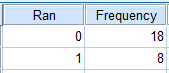If the data are available only as a frequency table, and not as a column with values as shown above, you will have to enter the data as a weighted table, with one categorical (numeric) variable and a count (integer) variable containing the frequency. The table will have two rows, one for each of the two values. See instructions for applying the frequency weight for your table.See example output

### Chi square (X²)  goodness of fit test

Analyze > Nonparametric Tests > Legacy Dialogs > Chi Square

#### Data set-up: Option 1Requires one categorical variable with values of expected frequencies. In SPSS, the variable must be stored as a numeric type and either nominal or scale measure.

#### Data set-up: Option 2If the data are available only as a frequency table, and not as a column with values as shown above, you will have to enter the data as a weighted table, with one categorical variable and a count (scale) variable containing the frequency. The table will have two rows, one for each of the two values. See instructions for applying the frequency weight for your table.See example output

## Tests for analyzing relationship between two categorical variables

### Chi square (X²) contingency test

Analyze > Descriptive Statistics > Crosstabs

#### Data set-up: Option 1Requires two categorical variables, with two or more possible values.

#### Data set-up: Option 2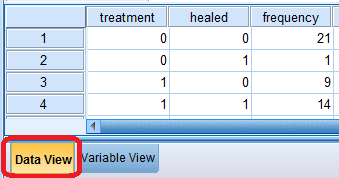If the data are available only as a frequency table, and not as a column with values as shown above, you will have to enter the data as a weighted table, with two categorical (numeric) variables and a count (integer) variable containing the frequency. The table will have one row for each possible combination of the two categorical variables; for example, if both categorical variables have three possible values, there will be 9 (3 x 3) rows. See instructions for applying the frequency weight for your table.See example output

### Fisher's exact test

Analyze > Descriptive Statistics > Crosstabs

#### Data set-up: Option 1Requires two categorical variables with two possible values each.

#### Data set-up: Option 2If the data are available only as a frequency table, and not as a column with values as shown above, you will have to enter the data as a weighted table, with one categorical variable and a count (integer) variable containing the frequency. The table will have four rows, one for each combination of the two variables. See instructions for applying the frequency weight for your table.See example output

## Tests for analyzing a single numerical variable

### One-sample t-test

Analyze > Compare Means > One-Sample T TestRequires one normally distributed numerical variable and a hypothesized mean. See instructions for checking for normality.See example output

### Sign test for median

Analyze > Nonparametric Tests > Legacy Dialogs > BinomialRequires one numerical variable and a hypothesized median. The numerical variable does not need to be normally distributed.See example output

## Tests with a numerical response variable and explanatory categorical variable(s) (Parametric)

### Two-sample t-test

Analyze > Compare Means > Independent-Samples T TestRequires one normally distributed, numerical variable and one grouping variable with two values. The grouping variable may be numeric-type or string-type. See instructions for checking for normality.See example output

### Paired t-test

Analyze > Compare Means > Paired-Samples T Test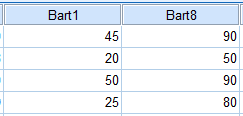Requires two numerical variables that are paired. Paired samples are matched in some way; often they represent the same object or respondent tested at different points in time.See example output

### One-way ANOVA and Tukey-Kramer test

Analyze > Compare Means > One-Way ANOVA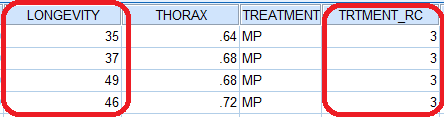Requires one normally distributed, numerical response variable and one categorical grouping variable with two or more values. See instructions for checking for normality. Note that the grouping variable must be numeric. If it is a string variable, like TREATMENT in the image below, you will need to create a  numeric-type variable using Automatic Recode.See example output

### Welch's t-test

Analyze > Compare Means > One-Way ANOVARequires one numerical response variable and one grouping variable with two values. See instructions for checking for normality. Note that the grouping variable must be numeric. If it is a string variable, like TREATMENT in the image below, you will need to create a numeric-type variable using Automatic Recode.See example output

### Multiway ANOVA

Analyze > General Linear Models > UnivariateRequires one normally distributed numerical response variable and two categorical grouping variables with two or more values. See instructions for checking for normality. Note that the grouping variable must be numeric. If it is a string variable, like TREATMENT in the image below, you will need to create a numeric-type variable using Automatic Recode.See example output

## Tests with a numerical response variable and an explanatory categorical variable (Non-parametric)

### Mann-Whitney U-test

Analyze > Nonparametric > Legacy Dialogs > 2 Independent Samples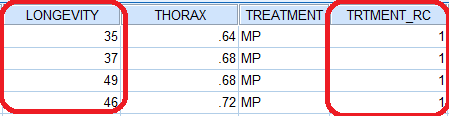Requires one numerical or ordinal variable, and one grouping variable with two values. The grouping variable must be numeric. If it's not numeric, use Automatic Recode to create a new numeric variable. The continuous variable does not need to be normally distributed.See example output

### Kruskal-Wallis Test

Analyze > Nonparametric > Legacy Dialogs > K Independent SamplesRequires one numerical or ordinal variable, and one grouping variable with two or more values. If it is a string variable, like TREATMENT in the image below, you will need to create a a numeric variable using Automatic Recode.See example output

## Tests for analyzing the relationship between numerical variables

### Simple linear regression

Analyze > Regression > Linear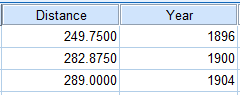Requires two numerical variables. See also instructions for creating regression plots.See example output

### Linear correlation

Analyze > Correlate > Bivariate

Requires two numerical variables. See setup for Simple linear regression above.See example output

### Spearman's rank correlation

Analyze > Correlate > Bivariate.

Requires two numerical variables. See setup for Simple linear regression above.See example output

### General linear model

Analyze > General Linear Model > Univariate

Requires one numerical dependent variable and any combination of independent numerical or categorical numeric variables.See example output